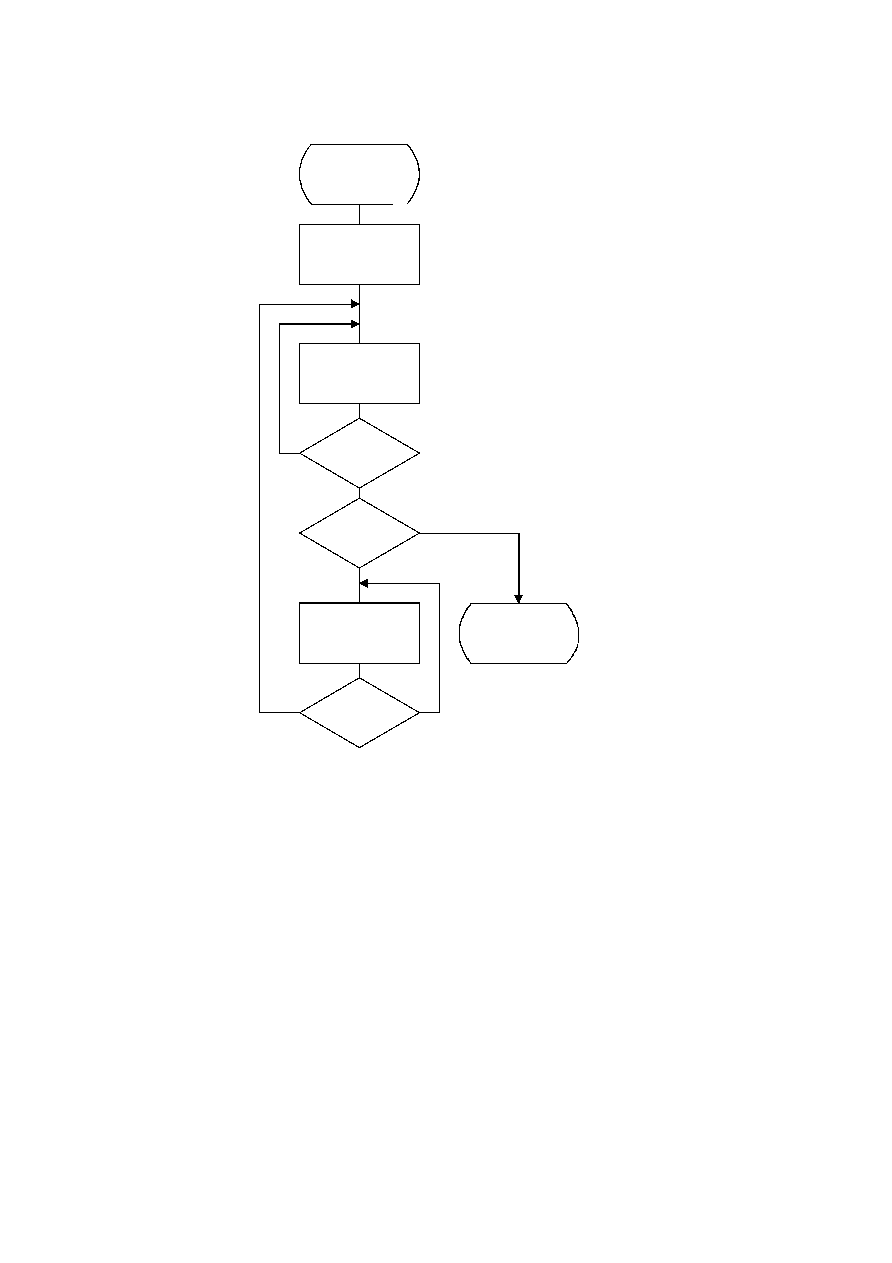ISO/IEC 10918-1 : 1993(E)
A Huffman code table, HUFFCODE, containing a code for each size in HUFFSIZE is generated by the procedure in
Figure C.2. The notation "SLL CODE 1" in Figure C.2 indicates a shift-left-logical of CODE by one bit position.
TISO1010-93/d037
Generate_code_table
K = 0
CODE = 0
SI = HUFFSIZE(0)
HUFFCODE(K) = CODE
CODE = CODE + 1
K = K + 1
Yes
No
HUFFSIZE(K) = SI
?
Yes
No
HUFFSIZE(K) = 0
?
Done
No
Yes
CODE = SLL CODE 1
SI = SI + 1
HUFFSIZE(K) = SI
?
Figure C.2 ­ Generation of table of Huffman codes
Figure C.2 [D37], = 16.5 cm = 645.%
Two tables, HUFFCODE and HUFFSIZE, have now been generated. The entries in the tables are ordered according to
increasing Huffman code numeric value and length.
The encoding procedure code tables, EHUFCO and EHUFSI, are created by reordering the codes specified by
HUFFCODE and HUFFSIZE according to the symbol values assigned to each code in HUFFVAL.
52
CCITT Rec. T.81 (1992 E)